# Power Factor

By on

Power factor is the ratio between the real power (P in kW) and apparent power (S in kVA) drawn by an electrical load.

The reactive power (Q in kVAr) causes the real and apparent power to be displaced from each other.  Reactive power provides the necessity for electric and magnetic fields to enable the power system to work.

In addition to being the ratio of real power to apparent power, the power factor can also be express as the cosine of the angle between the two.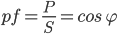If the reactive power of the load is inductive, the real power will lag the apparent power and the power factor will be lagging.  If the reactive power is capacitive the power factor will be leading.

In it's simplest form power factor, can also be considered a measure of the useful work obtained from a power system

Three phase power factor and single phase power factor follow the same concepts

For a theoretical discussion of power factor, see the section on Complex Power on the Alternating Current Circuits note.

### Typical Power Factors

Average Power Factor values for the most commonly used plant, equipment and appearances:

plant and appliances cos φ tan φ
induction motor - loaded at 0% 0.17 2.80
induction motor - loaded at 25% 0.55 1.52
induction motor - loaded at 50% 0.73 0.94
induction motor - loaded at 75% 0.80 0.75
induction motor - loaded at 100% 0.85 0.62
lamps incandescent 1.0 0
lamps fluorescent (uncompensated) 0.5 1.73
lamps fluorescent (compensated) 0.93 0.39
lamps discharge 0.4 to 0.6 2.29 to 1.33
oven resistance elements 1.0 0
oven induction heating (compensated) 0.85 0.62
oven dielectric heating 0.85 0.62
resistance type soldering machines 0.8 to 0.9 0.75 to 0.48
arc-welding fixed 1-phase 0.5 1.73
arc-welding motor-generator set 0.7 to 0.9 1.02 to 0.48
arc-welding transformer-rectifier set 0.7 to 0.8 1.02 to 0.75
arc furnace 0.8 0.75

Source: Groupe Schneider - Electrical installation guide
(According to IEC International Standards), 1996

### Harmonic Distorted Waveforms

Example - true power factor

A load is operating with a displacement power factor of 0.875 and THD of 13.4%.  What is the true power factor?

The distortion power factor is given by:

Resulting in a true power factor of: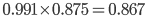Power factor as set up above assumes a sinusoidal wave form.  In a modern power system with the growth of power electronic devices, the waveform is generally not sinusoidal.  In this instance the definition of power factor becomes a little more complicated.

• displacement power factor - is the power factor of the 50 Hz fundamental for a harmonic distorted waveform
• distortion power factor - is the amount the displacement power is reduced due to harmonic content
• true power factor - is the actual power factor, taking into account the harmonic distortion

In a non-sinusoidal waveform the harmonic content reduces the power delivered to the load.  True power factor will always be less than the displacement power factor. The ratio of the true power factor to the displacement power factor is the distortion power factor.  For purely sinusoidal waveforms the distortion power factor is always 1.

If the total harmonic distortion is know, then the distortion power factor can be found from: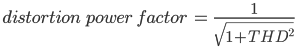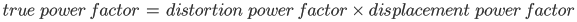## Power Factor Correction

Calculation of Required kVAr

Existing Situation is defined as load P in kW with power factor pf1  (assume lagging)

Leading  to, complex power S1 in kVA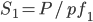Current Phase angle φ1 is given by: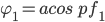The desired situation is defined as new power factor pf2
New Phase angle φ2 is give by: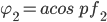Numerical Example

Existing Situation: P = 450 kW pf1 = 0.83
S1 = 450/0.83 = 542 kVA

φ1 = cos-1(0.83) = 33.9 degrees

Desired Situation: pf2 = 0.95
φ2 = cos-1(0.95) = 18.2 degrees

Calculation Results:
Q2 = 450 * (tan(33.9)-tan(18.2)) = 154 kVAr

S2 = 450/0.95 = 473 kVA (12.7% reduction)

By improving the power factor, power supply authorities need to generate less reactive power and power distribution systems become more efficient.  Power supply authorities often charge a penalty for power factor and it can be financially beneficial for the owner of equipment to provide systems to improve their power factor.

Power factor correction is typically carried out by the addition of capacitors – creating reactive power 180o out of phase with that created by the loads (typically inductive).

Power factor correction may be applied as bulk correction at the main plant switchboard or installed locally at each load.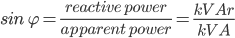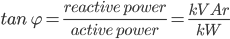If the kW is to remain constant then:

and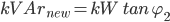giving: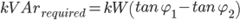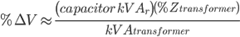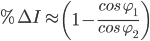### Power Factor Correction Tool

The calculation of the amount of reactive power required to achieve a given improvement is relatively easy to calculate.

To assist in the calculation of required power factor compensation we have added a power factor correction calculation tool to our site. See the links below for details.

More interesting Notes:Steven McFadyen

Steven has over twenty five years experience working on some of the largest construction projects. He has a deep technical understanding of electrical engineering and is keen to share this knowledge. About the author

#### View 2 Comments (old system)

1.Sinnadurai says:
7/11/2012 11:56 AM

Why is the pfc disconnected when a standby generator feeds the load?

•Steven says:
7/11/2012 12:38 PM

It is not the power factor correction per say, but the possibility of leading power factors which are not good for generators. Generators have a limited ability to absorb real or reactive power (as opposed to normal power systems which are usually much bigger than any load).

Best thing is to manage the power factor well. Switching off seems an easy approach, but you need to be careful that the actual power factor is not so low as to exceed the generator kVA rating.

Comments are closed for this post:
• have a question or need help, please use our Questions Section
• spotted an error or have additional info that you think should be in this post, feel free to Contact Us

IEC 61439 - The Switchgear Standard

The new standard IEC 61439 replaces the old 60439. Compared to the old standard, the new 61439 is a more clearly defined and takes into account the assembly...

IEC 60287 Current Capacity of Cables - An Introduction

IEC 60287 "Calculation of the continuous current rating of cables (100% load factor)" is the International Standard which defines the procedures and equations...

Cable Insulation Properties

Cable insulation is used to provide electrical separation between conductors of  a cable.  During the historical development of cables, numerous types...

What is an Open Delta Transformer

In three phase systems, the use of transformers with three windings (or legs) per side is common.  These three windings are often connected in delta or...

Three Phase Power Simplified

A single phase system is perhaps the most common type of system most people are familiar with. This is what people have in their homes and what appliances...

Paths of Flight

GE have put together a time-lapse video shown flight take-off and landings at some airports. An interesting view:

Tips for a better Low Voltage Protection Discrimination Study

Carrying out a protection system discrimination study is critical to ensure the correct functioning of  the electrical system in the event of faults. ...

Voltage Levels to IEC 60038

The standard aims to consolidate AC and traction voltages within the industry and defines the following bands: band 1 - A.C. systems 100 V to 1...

Three Phase Current - Simple Calculation

The calculation of current in a three phase system has been brought up on our site feedback and is a discussion I seem to get involved in every now and...

IEC 60287 Current Capacity of Cables - Rated Current

In the previous note we looked at the approach taken by the standard to the sizing of cables and illustrated this with an example.  We then looked at one...## Have some knowledge to share

If you have some expert knowledge or experience, why not consider sharing this with our community.

By writing an electrical note, you will be educating our users and at the same time promoting your expertise within the engineering community.

To get started and understand our policy, you can read our How to Write an Electrical Note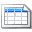Click here to view the notes list in a table formatClick here to view the notes indexed by tag